Students can access the CBSE Sample Papers for Class 12 Chemistry with Solutions and marking scheme Term 2 Set 7 will help students in understanding the difficulty level of the exam.

## CBSE Sample Papers for Class 12 Chemistry Standard Term 2 Set 8 with Solutions

Max. Marks: 35
Time: 2 Hours

General Instructions:
Read the following instructions carefully:

• There are 12 questions in this question paper with internal choice.
• Section A – Q. No. 1 to 3 are very short answer questions carrying 2 marks each.
• Section B – Q. No. 4 to 11 are short answer questions carrying 3 marks each.
• Section C- Q. No. 12 is case-based question carrying 5 marks.
• All questions are compulsory.
• Use of log tables and calculators is not allowed.

Section – A

Question 1.
If the concentration is expressed in mol L-1 units and time in seconds, what would be the unit of K*
(a) For a zero-order reaction
(b) For a first order reaction
(c) Why molecularity of a reaction cannot be zero?
(a) For zero order reaction unit of K is mol L-1 sec-1.
(b) For first order reaction unit of K is sec’-1.
(c) Molecularity is the number of given reactant molecules or atoms that are colliding in the elementary reaction. Thus, a minimum of one reactant molecule, atom or ion is required to initiate a chemical
reaction. Hence, molecularity cannot be zero.

Question 2.
Complete the following equations: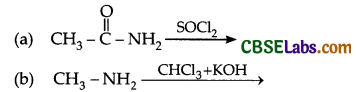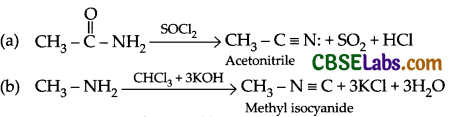Question 3.
Predict the product of the following reactions: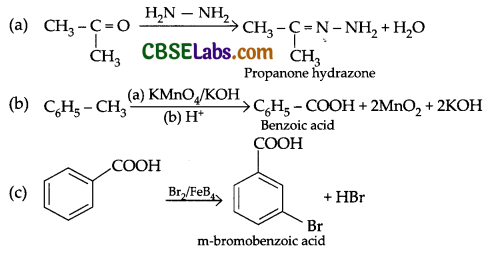Section – B

Question 4.
(a) Name the isomerism shown by the complex [C0(NH3)5(NO2)]Cl2.
(b) What type of bond is present between metal and carbon in metal carbonyls?
(c) [FeF6]3- has five unpaired electrons while [Fe(CN)6]3-has only one unpaired electron. Explain.
OR
The crystal field theory assumes that the interaction between the metal ion and ligand is purely electrostatic. When ligands approach central metal atom/ion the five degenerated orbitals of the central metal atom become differential. In a complex, the central metal atom or ion is surrounded by various atoms or groups of atoms called ligands.
(a) What is the type of bond in metal complex according to crystal field theory?
(b) What is the number of ligands in Cu2 [Fe(CN)6].
(c) Which one is lower energy set between t2g and eg, after splitting of five degenerate orbital or metal atom/ion on complexation in tetrahedral complex?
(a) Linkage isomerism occurs in complex compounds with ambidentate ligands like NO2, SCN, CN, S2O3-2.
These ligands have two donor atoms but at a time only one atom is directly linked to central metal atom of the complex. Linkage isomerism is shown by [Co(NH3)5(NO2)] Cl2. The isomers are [Co(NH3)5NO2]Cl and [Co(NH3)5ONO]Cl2.

(b) In a metal carbonyl, the metal-carbon bond possesses both σ and π character. Here M – C π bond is formed by the donation of lone pair of electrons from carbonyl carbon to the vacant orbital of metal. M – C π bond is formed by the back donation of electrons from a filled d-orbital of metal into the vacant antibondirig n orbital of carbon monoxide (Carbonyl ligand).

(c) In [FeF6]3-Fe(III) has got 3d5 systgem. As F is a weak field ligand, according to crystal field theory (CFT) electronic arrangement will be as follows:Hence, it has 5 unpaired electrons.
In [Fe(CH)6]3- Fe(III) has got 3d5 system. As CN is a strong field ligand, according to CFT electronic
the arrangement will be as follows: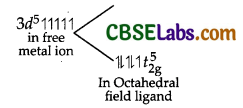Hence, it has only one unpaired electron.
OR
(a) Ionic bond or electrostatic bond.
(b) Number of ligands = 6.
(c) Lower energy set = eg.

Ionic Strength Calculator is a free online tool that displays the ionic strength for the given concentration and valency.

Question 5.
Following ions are given:
Cr2+, Cu2+, Cu+, Fe2+, Fe3+, Mn3+
Identify the ion which is
(a) A strong reducing agent.
(b) Unstable in aqueous solution.
(c) A strong oxiding agent.
Give suitable reason in each case.
OR
Compare the general characteristics of the first series of the transition metals with those of the second and third series metals in the respective vertical columns. Give special emphasis on the following points:
(a) Electronic configurations
(b) Oxidation states
(c) Ionisation enthalpies.
(a) Cr2+’ is a stronger reducing agent because it can lose one of its electron to become more stable Cr3+
in which the t2g level of d-orbital is half filled arid eg level is empty.
(b) Cu+ is unstable in aqueous solution because it disproportionates in water to form Cu2+ and Cu.
(c) Mn3+ is a stronger oxidising agent because it has 4 electrons in its valence shell and when it gains
one electron to form Mn2+, it results in the half-filled (d5) configuration that has extra stability.
OR
(a) In the 1st, 2nd and 3rd transition series, the 3d, 4d and 5d orbitais are respectively filled.
We know that elements in the same vertical column generally have similar electronic configurations.
In the first transition series, two elements show unusual electronic configurations:
Cr (24) = 3d54s1 (Because of extra stability of half filled and fully filled orbitais)
Cu (29) = 3d10 4s1
Similarly, there are exceptions in the second transition series. These are:
Mo(42) = 4d55s1
Te(43) = 4d65s1
Ru(44) = 4d75s1
Rh(45) = 4d85s1
Pd(46) = 4d105s0
Ag(47) = 4d105s1
There are some exceptions in third transition series as well. These are:
W(74) = 5d46s2
Pt(78) = 5d96s1
Au(79) =5d106s1

As a result of these exceptions, it happens many times that the electronic configurations of the elements present in the same group are dissimilar.
(b) In each of the three transition series the number of oxidation states shown by the elements is the maximum in the middle and minimum at the extreme ends.

However, +2 and +3 oxidation states are quite stable for all elements present in the first transition series.
All metals present in the first transition series form stable compounds in the +2 and +3 oxidation states.
The stability of the +2 and +3 oxidation states decreases in the second and the third transition series,
wherein higher oxidation states are more common.

For example, chromate ion (CrO4)2- is strong oxidant while molybdate (MoO4)2- and tungstate (WO4)2-
are stable. For example: WCl6, ReF7, RuO4, etc., are stable but no such compound of 1st transition series exists.

(c) In each of the three transition series, the first ionisation enthalpy increases from left to right. The first ionisation enthalpies of the third transition series are higher than those of the first and second transition series. This occurs due to the poor shielding effect of 4f electrons in the third transition series. The removal of one electron alters the relative energies of 4s and 3d orbitals. Hence, there is marginal and irregular increase in ionisation enthalpies.

Question 6.
(a) How would you account for the fact that the transition elements have high enthalpies of atomisation?
(b) Name the catalyst used in Ostwald’s process for the manufacturing of nitric acid.
(c) Transition metals generally form coloured compounds. Explain why?
(a) Transition elements have large number of unpaired electrons in their atoms due to which they have stronger interatomic interactions and hence stronger bonding between atoms, leading to high
atomisation enthalpies.

(b) Platinised asbestos is used as catalyst in the Ostwald’s process for the manufacturing of nitric acid.

(c) Transition metals generally form coloured compounds because they generally possess one or more unpaired electrons. When visible light falls on a transition metal compound or ion, the unpaired electrons present in the lower energy d-orbital get promoted to high energy d-orbitals, called d-d transition, due to the absorption of visible light. Since the energy involved in d-d transition is quantised, only a definite wavelength gets absorbed, remaining wavelengths present in the visible region got transmitted. Therefore, transmitted light shows some colour complementary to the absorbed colour.

Question 7.
Write one difference in each of the following:
(a) Multimolecular colloid and Associated colloid
(b) Coagulation and Peptization
(c) Lyophobic sol and Lyophilic sol
(a) Multìmolecular colloids: It consists of aggregates of a large number of atoms or smaller molecules. Its size is in the range of 1-1000rim. Associated colloids: At low concentration, they act as strong electrolytes whereas at high concentrations form aggregates or micelles

(b) Coagulation is the process of aggregating together the colloidal particles so as to change them into large sized particle by the addition of excess of an electrolyte whereas peptisation is the process of converting a fresh precipitate into colloidal particles by adding small amount of suitable electrolyte.

(c) Lyophobic colloidal sols are not hydrated and have weak affinity with the dispersion medium whereas lyophilic colloidal sols are heavily hydrated and have strong affinity with the dispersion medium.

Question 8.
(a) A galvanic cell consists of metallic zinc plate dipped in 0.1 M zinc nitrate solution and a lead plate dipped in 0.02 M lead nitrate solution. Write chemical equation for the electrode reaction and calculate the e.m.f. of the cell at 25°C.
E0Zn2+/Zn = – 0-76 V, E°pb2+/Pb = – 0.13 V
(b) Why does the conductivity of a solution decrease with dilution?
(c) What is the use of salt bridge in an electrochemical cell?
(a) The half cell reactions can be written as:
Pb2++ 2e → Pb, E°pb2+/Pb=-0.13V
Zn2+ + 2e → Zn, E°Zn2+ /Zn =-0.76 V
So, E°cell = E°cathode — E°anode
=-0.13V-(-0.76V)
=-0.13+0.76 -0.63V
Now, overall reaction:
Zn+Pb2+ → Zn2+Pb;Ecell=?

Ecell = E°cell – $$\frac{0.0591}{2} \log \left[\frac{\mathrm{Zn}^{+2}}{\mathrm{~Pb}^{+2}}\right]$$
= 0.63 – $$\frac{0.06}{2} \log \left(\frac{0.1}{0.02}\right)$$
=0.63-0.03 log5
=0.63-0.03 x 0.6910
= 0.63 — 0.02 = 0.61V

(b) The conductivity of a solution is the conductance of ions present in a unit volume of the solution. The number of ions (responsible for carrying current) decreases when the solution is diluted. As a result, the conductivity of a solution decreases with dilution.

(c) The purpose of the salt bridge is to act as a source of spectator ions that can migrate Into each of the half cells to preserve electrical neutrality.

Question 9.
(a) An organic compound X has molecular formula C5H10O. It does not reduce Fehling’s solution but
forms a bisulphate compound. It also gives positive iodoform test. What are possible structures of X? Explain your reasoning relating structure.
(b) Write IUPAC name of the following: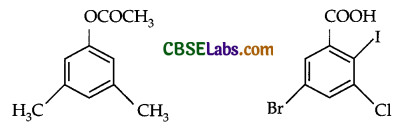OR
Predict the product: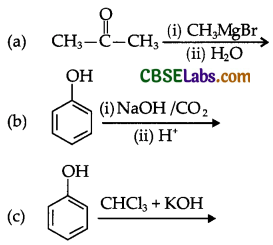(a) As the compound gives adduct with bisulphite, i.e., bisulphate compound, it contains a carbonyl group. But it does not reduce Fehling’s solution so it must be a ketone. Now, as this gives a positive test with iodoform, it is methyl ketone. So, based on the above information the following are the possible structures of the compound:Question 10.
Answer the following questions:
(a) What is Hinsberg reagent?
(b) Why do primary amines have higher boiling points than tertiary amines?
(c) Why are aliphatic amines stronger bases than aromatic amines?
(a) Benzenesulphonyl chloride (C6H5 – SO2Cl) is known as the Hinsberg reagent.

(b) Primary amines are involved in intermolecular association due to H-bonding. While 3° amines do not have intermolecular association due to lack of H-bonding. Thus, Molecular association of primary amine leads to the increase of its effective molar mass and thus, 1° amine has higher boiling point than 3° amine.

(c) Due to the -I effect of the benzene ring, the electrons on the N-atom are involved in resonance and are available as base. Therefore, the electrons on the N-atom in aromatic amines cannot be donated easily. This is the reason that aliphatic amines are stronger bases than aromatic amines.

Question 11.
Predict the product:Or
(a) Arrange the following in increasing acidic strength: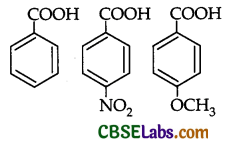(b) Distinguish between:
(i) Acetone and formaldehyde
(ii) C6H5COCH3 and C6H5CH2 – CHOSection – C

Question 12.
Read the passage given below and answer the questions that follow:
The term order of a reaction is the sum of exports of concentration terms appearing in the rate equation. It is an experimentally determinable quantity. It may be whole number, fraction, zero on even negative. Knowledge of order does not require knowledge of mechanism. Order of a ‘reaction’ may change with change in experimental condition namely pressure, temperature etc.

A 1st order reaction is a reaction whose rate-determining step (r.d.s) involves only one molecule. Thus the step is A → Product.
A 2nd order reaction may be of two types:
2 A→ Y Product
or A + B → Product.
(a) Give the unit of rate constant of a reaction.
(b) In what condition order and molecularity of a reaction becomes equal.
(c) In which type of reaction order and molecularity are different.
(d) Write expression for rate constant of first-order reaction. Mention unit of rate constant of a first-order reaction.
OR
(d) Show that half-life period of a first-order reaction is independent of initial concentration of the reactant.
= $$\frac{\mathrm{mol} \cdot \mathrm{L}^{-1}}{s} \times \frac{1}{\left(\mathrm{~mol}, \mathrm{~L}^{-1}\right)}$$ = s-1
k= $$\frac{2.303}{t} \log \frac{[\mathrm{R}]_{0}}{[\mathrm{R}]}$$
At t1/2‘[R] = $$\frac{[\mathrm{R}]_{0}}{2}$$